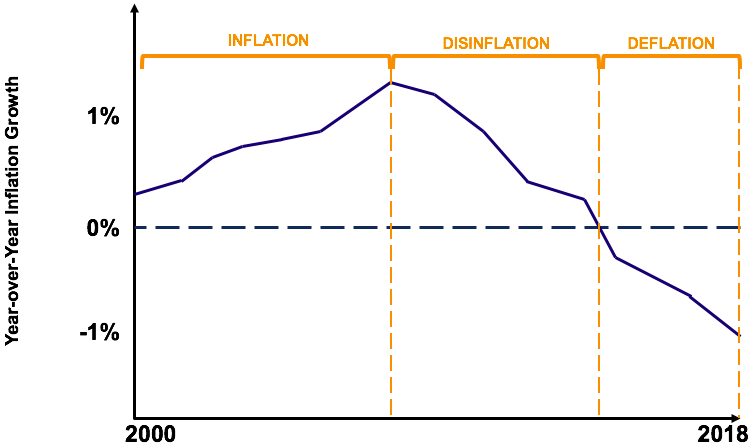# Disinflation

A slowdown in the rate of inflation

## What is Disinflation?

Disinflation is used to describe the slowing of price inflation. In other words, it is a decrease in the rate of inflation. The term should not be confused with deflation, which is used to describe a negative inflation rate.

### Disinflation vs. Deflation

The terms disinflation and deflation are commonly mixed up. The definitions of the two terms are contrasted below followed by a diagram illustrating inflation, disinflation, and deflation:

Disinflation: A situation where inflation increases at a slower rate.

Deflation: A situation where inflation is negative (i.e., a decrease in the prices of goods and services in the economy).As illustrated by the graph:

• Periods of rising inflation are termed inflation
• Periods of decreasing inflation are termed disinflation
• Periods of negative inflation are termed deflation

An easy way to quickly differentiate between deflation and disinflation is that the former is always negative while the latter is positive but decreasing. As shown by the graph, decreasing year-over-year inflation is termed disinflation while a negative inflation rate is termed deflation.

### Primary Causes of Disinflation

It is important to note that inflation is caused by an increase in the supply of money in the economy. Therefore, a slowdown in the economy’s money supply through a tighter monetary policy is an underlying cause of disinflation.

In some cases, a slowdown in the rate of inflation can also arise during an economic recession. For example, in a recession, businesses may refrain from increasing their price levels to gain more customers (causing disinflation).

### CPI as a Measure of Determining Disinflation

A widely used measure of inflation is called the Consumer Price Index (CPI). The CPI measures the changes in the price level of consumer goods and services and is one of the most closely watched economic statistics among investors and the Federal Reserve. The percentage change in the CPI is used as a measure of inflation.

For example, assume the CPI was as follows for 2016, 2017, and 2018, respectively:

• 2016 CPI: 101.7
• 2017 CPI: 102.3
• 2018 CPI: 102.6

If we take the percentage change of CPI from each year, the annual inflation rate can be determined. Using 2016 as the base year, the inflation for 2017 was 0.6% (102.3/101.7 – 1) and the inflation for 2018 was 0.3% (102.5/102.3 – 1). Since the inflation rates for 2017 and 2018 were 0.6% and 0.3%, respectively, it demonstrates a period of disinflation.

### Example of Disinflation

The following are the CPI’s of a hypothetical economy for several years. As an analyst, your manager wants to know whether the economy experienced either inflation, disinflation, or deflation from 2013 to 2017:

• 2013 CPI: 100
• 2014 CPI: 101
• 2015 CPI: 102.1
• 2016 CPI: 102.9
• 2017 CPI: 103.3

To determine whether the economy experienced either inflation, disinflation, or deflation, we first must determine the inflation over each year by determining the annual change in CPI between years. Doing so gives us the inflation rate for each time period. Using 2013 as a base (starting) year:

• Inflation from 2013 to 2014: 101/100 – 1 = 1%
• Inflation from 2014 to 2015: 102.1/101 – 1 = 1.1%
• Inflation from 2015 to 2016: 102.9/102.1 – 1 = 0.8%
• Inflation from 2016 to 2017: 103.3/102.9 – 1 = 0.4%

From 2013 to 2015, the economy experienced rising inflation. Inflation was 1% from 2013 to 2014 and 1.1% from 2014 to 2015.

From 2015 thereon, the country experienced disinflation. Inflation was 0.8% from 2015 to 2016 and further decreased to 0.4% from 2016 to 2017.